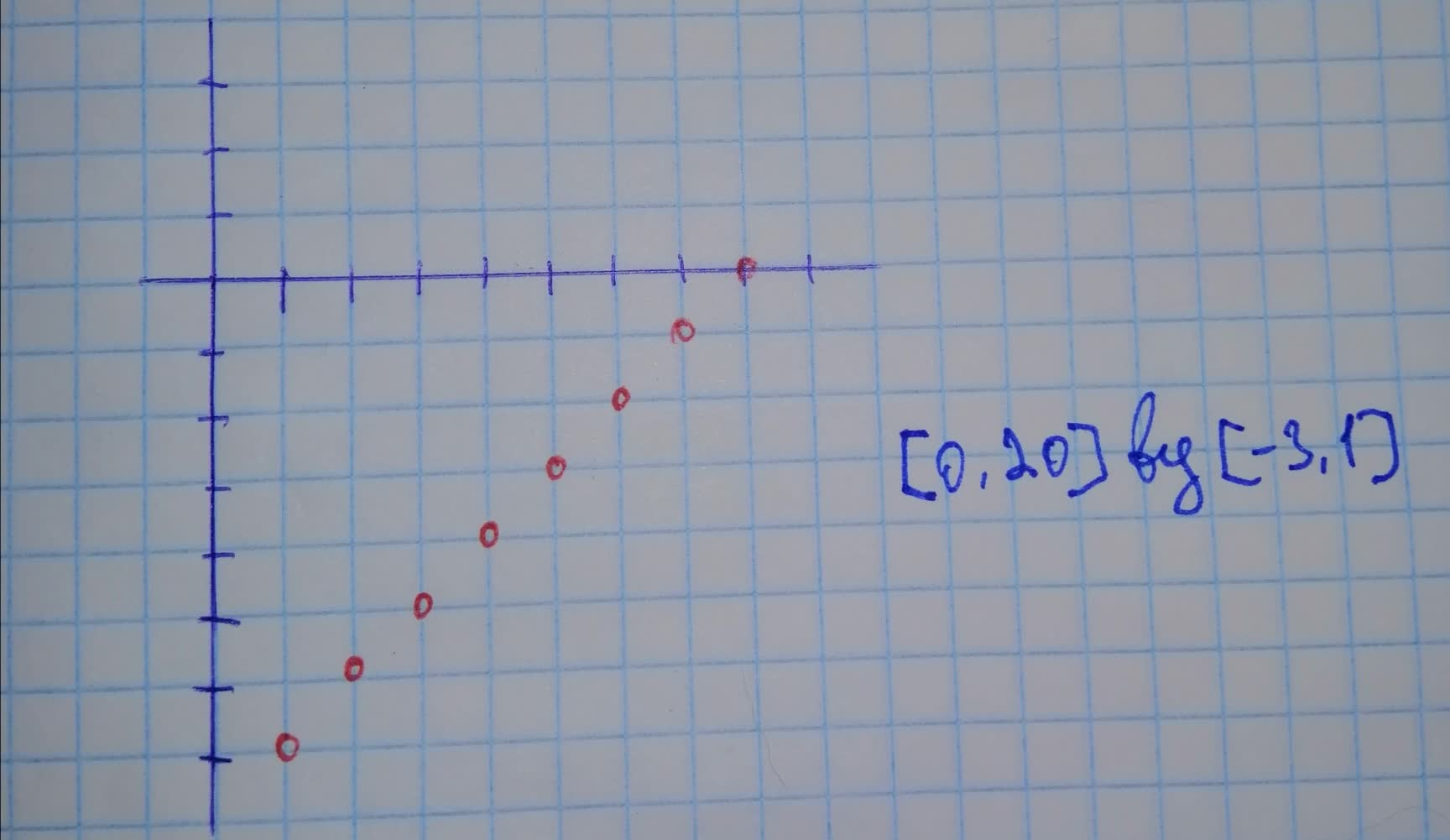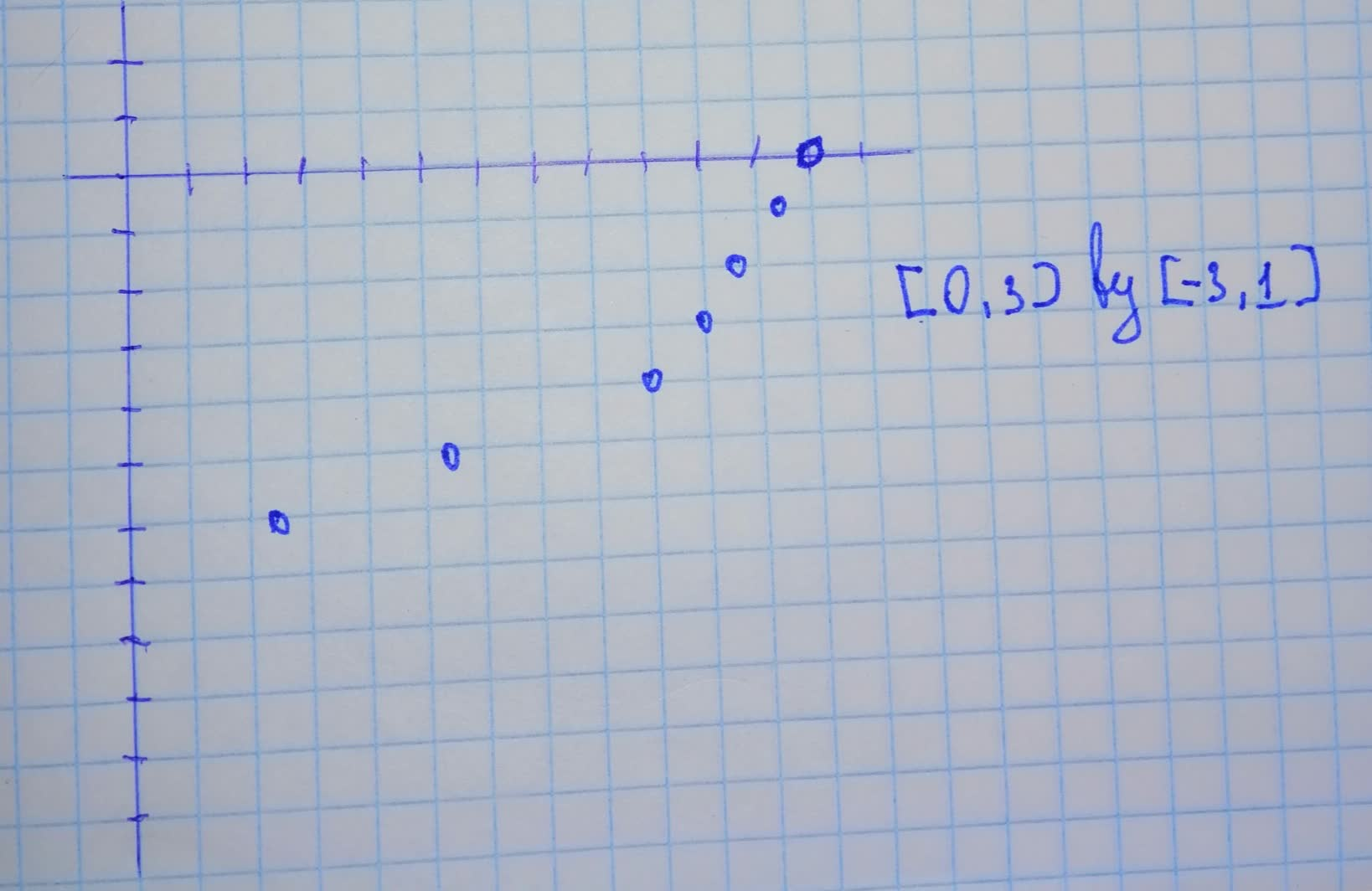# Data points (x,\ y) \begin{array}{|c|c|}\hline x & & 2 & 4 & 6 & 8pamangking8 2021-11-16 Answered
Data points $$\displaystyle{\left({x},\ {y}\right)}$$
$\begin{array}{|c|c|}\hline x & & 2 & 4 & 6 & 8 & 10 & 12 & 14 & 16 \\ \hline y & 0.08 & 0.12 & 0.18 & 0.25 & 0.36 & 0.52 & 0.73 & 1.06 \\ \hline \end{array}$
Draw scatter plots of $$\displaystyle{\left({x},\ {\ln{{y}}}\right)}$$ and $$\displaystyle{\left({\ln{{x}}},\ {\ln{{y}}}\right)}$$

• Questions are typically answered in as fast as 30 minutes

### Plainmath recommends

• Get a detailed answer even on the hardest topics.
• Ask an expert for a step-by-step guidance to learn to do it yourself.Steven Arredondo

Step 1
In part (a), you already entered the x-coordinates under $$\displaystyle{L}_{{{1}}}$$ and the y-coordinates under $$\displaystyle{L}_{{{2}}}$$ in your graphing calculator.
Enter $$\displaystyle{L}_{{{3}}}={\ln{{\left({L}_{{{2}}}\right)}}}$$ into your graphing calculator so $$\displaystyle{L}_{{{3}}}$$ will fill in the values of $$\displaystyle{\ln{{y}}}$$ for you. Then go to STAT PLOT, choose Plot2, and change YList to $$\displaystyle{L}_{{{3}}}$$. Turn off Plot1 and turn on Plot2. Adjust the window to an appropiate veiwing rectangle and then press GRAPH:Step 2
Enter $$\displaystyle{L}_{{{4}}}={\ln{{\left({L}_{{{1}}}\right)}}}$$ into your graphing calculator so $$\displaystyle{L}_{{{4}}}$$ will fill in the values of $$\displaystyle{\ln{{x}}}$$ for you. Then go to STAT PLOT, choose Plot3, and change Xlist to $$\displaystyle{L}_{{{4}}}$$ and Ylist to $$\displaystyle{L}_{{{3}}}$$. Turn off Plot2 and turn on Plot3. Adjust the window to an appropriate viewing rectangle and then press GRAPH: